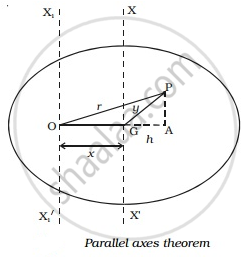# Prove the Theorem of Parallel Axes About Moment of Inertia - Physics

Sum

Prove the theorem of parallel axes about moment of inertia

#### Solution

The moment of inertia of a body about any axis is equal to the sum of its moment of inertia about a parallel axis through its centre of gravity and the product of the mass of the body and the square of the distance between the two axes.

Proof :

Let us consider a body having its centre of gravity at G as shown in Fig.. The axis XX′ passes through the centre of gravity and is perpendicular to the plane of the body. The axis X1X1′ passes through the point O and is parallel to the axis XX′ . The distance between the two parallel axes is x.Let the body be divided into large number of particles each of mass m . For a particle P at a distance r from O, its moment of inertia about the axis X1OX1′ is equal to m r 2

The moment of inertia of the whole body about the axis X1X1′ is given by,

I = Σ mr ???(1)

From the point P, drop a perpendicular PA to the extended OG and join PG.

In the ∆OPA,

OP 2 = OA2 + AP 2

r2 = x2 + 2xh + h2 + AP ???(2)

But from ∆ GPA,

GP 2 = GA2 + AP 2

2 = h 2 + AP 2  ..(3)

Substituting equation (3) in (2),

2 = x 2 + 2xh + y 2   ..(4)

Substituting equation (4) in (1),

I0   = Σ m (x2 + 2xh + y2)

= Σmx2 + Σ2mxh + Σmy2

= Mx2 + My2 + 2xΣmh

Here My2 = IG is the moment of inertia of the body about the line passing through the centre of gravity. The sum of the turning moments of all the particles about the centre of gravity is zero, since the body is balanced about the centre of gravity G.

Σ (mg) (h) = 0  (or)  Σ mh = 0 [since g is a constant]

equation (5) becomes, I0= Mx2 + IG

Thus the parallel axes theorem is proved.

Concept: Theorems of Perpendicular and Parallel Axes
Is there an error in this question or solution?